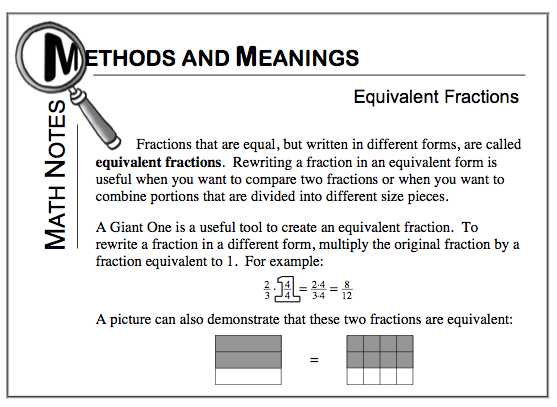### Home > CC2 > Chapter 1 > Lesson 1.2.8 > Problem1-137

1-137.

Rewrite each fraction in at least 2 different ways. Homework Help ✎

1. $\frac { 6 } { 9 }$

2. $\frac { 11 } { 12 }$

3. $\frac { 3 } { 8 }$

4. $\frac { 10 } { 7 }$

Answers may vary.

Refer to the Math Notes box below from Lesson 1.2.8 for help on rewriting equivalent fractions